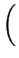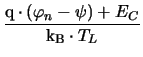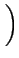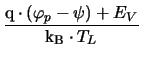Next: 3.2 Physical Parameters Up: 3.1 Sets of Partial Previous: 3.1.4 The Lattice Heat

## 3.1.5 The Constant Quasi-Fermi Potential Approximation

In unipolar devices like MOSFETs it is often possible to assume a constant quasi-Fermi potential for one carrier type. This implies that the current density for this carrier type vanishes.

The quasi-Fermi potential is used to calculate the local concentration of the considered carrier type. Each segment has a quasi-Fermi potential of its own which is determined by some contact. The advantage is that no continuity equation needs to be solved for this carrier system which reduces the simulation time. In case of a drift-diffusion equation set, the equation system size is reduced by about 1/3. In case of a hydrodynamic simulation the reduction of the equation system size is about 2/5 since the corresponding continuity equations and the energy flux equation are not solved, such that a five equation system reduces to a three equation system. This reference potential is then used to calculate the carrier concentrations in the respective segments. An example is an n-channel MOSFET, for which the holes in the substrate represent the minority carrier system. Since they contribute only marginally to the drain current, it is in many situations justified to ignore the hole current at all by assuming a constant quasi-Fermi level. The equations for the electron and hole concentration read

 n = NC . exp-(3.19) p = NV . exp. (3.20)Next: 3.2 Physical Parameters Up: 3.1 Sets of Partial Previous: 3.1.4 The Lattice Heat
Tibor Grasser
1999-05-31